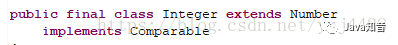``````

``````

blog.csdn.net/ysj4428/article/details/81195846## 一、为什么需要实现这个接口呢？

``````
String[] strArr = {"A","B","C","E","D"};
Arrays.sort(strArr);
for (String string : strArr) {
System.out.print(string+";");
}

``````

``````
A;B;C;D;E;

``````

``````
public final class String
implements Serializable, Comparable, CharSequence｛
...
｝

``````

``````
public class ComparableDemo{
public static void main(String[] args) {
Object[] objArray = {new Person(20,"jack"),new Person(17,"tom"),
new Person(27,"aj")};
for (Object object : objArray) {
System.out.print(object.toString());
}
Arrays.sort(objArray);
for (Object object : objArray) {
System.out.print(object.toString());
}

}
}

``````
``````
public class Person {
private Integer age;
private String name;
public Person(int age,String name) {
this.age = age;
this.name = name;
}
@Override
public String toString() {
return "Person [age=" + age + ", name=" + name + "]";
}

}

``````

``````
Person [age=20, name=jack]Person [age=17, name=tom]Person [age=27, name=aj]
Exception in thread "main" java.lang.ClassCastException: interfacedemo.Person cannot be cast to java.lang.Comparable
at java.util.ComparableTimSort.countRunAndMakeAscending(Unknown Source)
at java.util.ComparableTimSort.sort(Unknown Source)
at java.util.ComparableTimSort.sort(Unknown Source)
at java.util.Arrays.sort(Unknown Source)
at interfacedemo.ComparableDemo.main(ComparableDemo.java:18)

``````

## 二、接口简介

``````
{(x, y)|x.compareTo(y) &lt;= 0}。

``````

``````
{(x, y)|x.compareTo(y) == 0}。

``````

``````
{(x, y)|x.equals(y)}。

``````

## 三、实现该接口

``````
public class Person implements Comparable{
private Integer age;
private String name;
public Person(int age,String name) {
this.age = age;
this.name = name;
}
@Override
public String toString() {
return "Person [age=" + age + ", name=" + name + "]";
}
//进行方法重写
@Override
public int compareTo(Object o) {
Person p = (Person)o;
return this.age - p.age;
}

}

``````

``````
Person [age=20, name=jack]Person [age=17, name=tom]Person [age=27, name=aj]
Person [age=17, name=tom]Person [age=20, name=jack]Person [age=27, name=aj]

``````

### compareTo

``````
int compareTo(T o)

``````

o - 要比较的对象。

ClassCastException - 如果指定对象的类型不允许它与此对象进行比较。

``````

``````

``````

``````

上一篇一个复杂系统的拆分改造实践！

2021-04-04推荐一款神仙颜值的 Redis 客户端工具，开源啦

2021-04-04